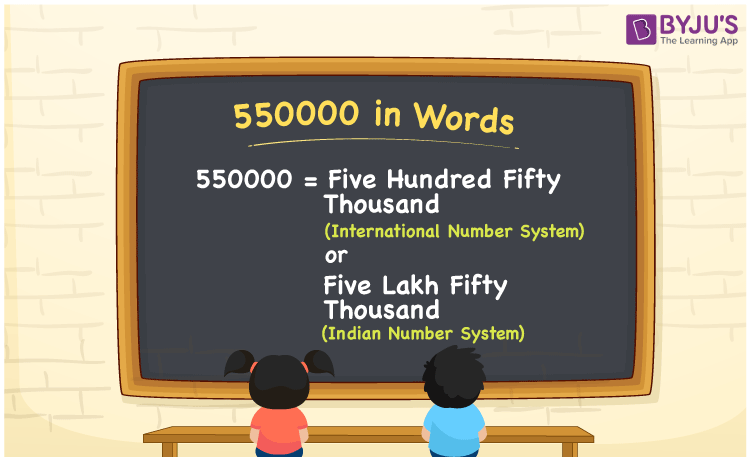# 550000 in Words

550000 in words can be written as Five Hundred Fifty Thousand in the International number system and as Five Lakh Fifty Thousand in the Indian number system. This article will help you write numbers in words using the English alphabet. Here, 550000 can be written in words in both the International and Indian number systems.

 550000 in words Five Hundred Fifty Thousand  (or) Five Lakh Fifty Thousand Five Hundred Fifty Thousand  (or) Five Lakh Fifty Thousand in Numbers 550000

## 550000 in English Words## How to Write 550000 in Words?

550000 in words – International number system

The table below provides you complete details of the place value chart for 550000 with respect to the International number system.

 Hundred Thousands Ten Thousands Thousands Hundreds Tens Ones 5 5 0 0 0 0

In expanded form it can be written as

5 x Hundred Thousand  + 5 x Ten Thousand + 0 x Thousand + 0 x Hundred + 0 x Ten + 0 x One

= 5 x 100000 + 5 x 10000 + 0 x 1000 + 0 x 100 + 0 x 10 + 0 x 1

= 500000 + 50000

= 550000

= Five Hundred Fifty Thousand

550000 in words – Indian number system

The place value chart for 550000 in the Indian system is provided here.

 Lakhs Ten thousands Thousands Hundreds Tens Ones 5 5 0 0 0 0

Here, ones = 0, tens = 0, hundreds = 0, thousands = 0, ten thousands = 5, lakhs = 5

That means, 5 lakhs and 5 ten thousands

= 5 lakhs + (5 × 10) thousands

= 5 lakhs + 50 thousands

= Five lakh Fifty thousand

550000 is a natural number that precedes 550001 and succeeds 549999.

550000 in words – Five Hundred Fifty Thousand (or) Five Lakh Fifty Thousand

Is 550000 an odd number? – No

Is 550000 an even number? – Yes

Is 550000 a perfect square number? – No

Is 550000 a perfect cube number? – No

Is 550000 a prime number? – No

Is 550000 a composite number? – Yes

## Frequently Asked Questions on 550000 in Words

### How do you write 550000 in words?

550000 can be written in words as “Five Hundred Fifty Thousand (or) Five Lakh Fifty Thousand”.

### Write Five Hundred Fifty Thousand (or) Five Lakh Fifty Thousand in numbers.

Five Hundred Fifty Thousand (or) Five Lakh Fifty Thousand can be written in numbers as 550000.

### Is 550000 an odd number?

No, 550000 is not an odd number as it is completely divisible by 2.